# Calculated Fields¶

Calculated Fields are used for two purposes:

• to calculate new sensor readings from other readings that are in the sensor database.
• to add sensor readings gathered from the Internet. The current implementation allows for acquisition of temperature and wind speed data from Internet weather services.

A Calculated Field uses the same editing form in BMON as a standard Sensor does. So, to add a Calculated Field, you follow the normal procedure for adding a Sensor, as described in Add Buildings and Sensors. The important configuration differences relative to a standard sensor are:

• For a Calculated Field, you need to create your own Sensor ID for the calculated field. The Sensor ID must be unique across the entire BMON set of Sensors. The Sensor ID needs to be 30 characters or less, using numbers and letters but no spaces.
• In the Sensor editing form, you need to make sure that the `Calculated Field` box is checked.

The BMON system processes Calculated Fields every one-half hour. If the calculated fields involve sensors that report more frequently than every half hour, multiple calculated sensor readings will be generated at each half-hour processing interval to match up with the frequency of the source sensors.

## Mathematical Calculated Fields¶

It is possible to create a calculated field by doing mathematical operations on one or more other sensor fields (a limit of five sensor fields can be involved in the calculation). For example, if you want to plot and analyze the difference in temperature between two sensors, you can create a new calculated “sensor” that is the difference in value between two other sensors. Below is a screen shot of how you would configure that calculated field.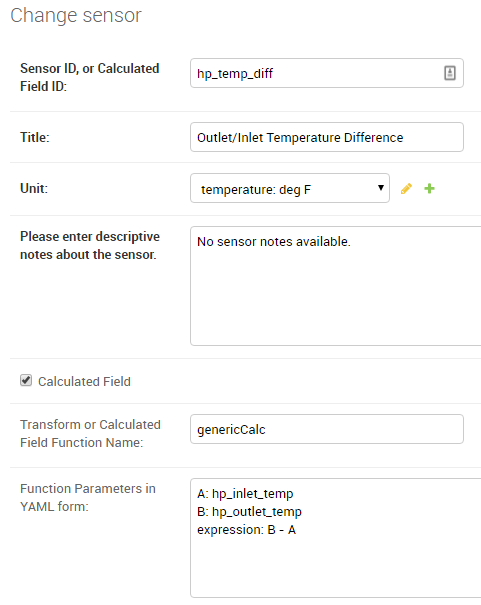This new calculated field has the Sensor ID of `hp_temp_diff`, and the values in that field are calculated as the difference between sensors with the IDs of `hp_outlet_temp` and `hp_inlet_temp`.

Not only are these calculated fields useful for plotting and analysis, they also extend the capabilities of the Alerting system, as described in Sensor Alerts. Complex alerts can be created by configuring a calculated field involving multiple sensors. Then, an Alert condition can be added to that calculated field. Thus, the Alert will depend on the state of multiple sensors, since multiple sensors feed the calculated field.

These calculated fields involving general mathematical expressions must have `genericCalc` entered in the “Transform or Calculated Field Function Name” box. This is the name of the function that allows for general calculations to be done using one or more existing sensor fields.

Remaining configuration of the calculated field occurs in the “Function Parameters in YAML form” input box. Each one of the possible function parameters is described below.

`A` (required: a Sensor ID)
The value of this parameter is the Sensor ID of the sensor that will be used as the `A` variable in the mathematical expression. At least one sensor needs to be involved in the calculation of the new field, so that is why the `A` parameter is required.
`B`, `C`, `D`, `E` (optional: a Sensor ID for each parameter used)
Four additional sensors can be involved in the calculation. Use any of the parameter names of B through E to give the Sensor IDs of those additional sensors involved in the calculated field.
`expression` (required: a math or boolean expression, described below)

Use this parameter to write out the math expression for the calculated field. You can use any of the sensor variables A through E that were included as parameters, and the values from these sensors will be used in the calculation. The expression must be a valid Python expression, and any of the functions in the Python `math` library can be included; see the math library documentation.

Here are some valid expressions:

An example of an expression using function from the Python math library, using three sensors as inputs (A, B and E):

```expression: 3.4 * A * sin(B) + sqrt(E)
```

An example of a Boolean expression. A `True` value from this expression is represented as a sensor value of 1.0 and a `False` value is represented as 0.0. An important note, when testing for equality with a Python expression, you must use a double equal sign (==) and not a single equal sign. The expression below will result in a calculated field value of 1.0 if Sensor A has a value equal to 1.0 and Sensor B has a value greater than 34.3; otherwise, the calculated value will be 0.0:

```expression: (A == 1.0) and (B > 34.3)
```
`averaging_hours` (optional: a number of hours, fractional hours allowed; default is no averaging)
If you include this parameter, it will cause sensor values to be averaged over the requested time interval before being used in the expression. So, if `averaging_hours` is 0.5, any sensor values will be averaged over half-hour intervals before they are used in the calculation.
`rolling_average` (optional: the value `True` or `False`, default is `False`)
When this parameter is `False`, the default, each averaging interval for the sensor values is distinct and does not overlap with other intervals. For example, if `averaging_hours` is set to 4.0, sensors will be averaged over 6 different periods across the day: Midnight to 4 am, 4 am - 8 am, etc. And, 6 calculated values will be produced corresponding to each one of those intervals. However, if `rolling_average` is set to `True`, a new calculated value will be computed for each and every timestamp that is present for sensor A. For example, if sensor A has a reading at 10:30 am, the `averaging_hours` is set to 2.0, and `rolling_average` is `True`, all sensors involved in the calculation will be averaged over the period of 8:30 am - 10:30 am (the 2 hours preceding sensor A’s timestamp), and a new calculated value will be created for that time interval. If another sensor A reading is present for 10:40 am, and new calculated value will be computed for the 8:40 am - 10:40 am period. So, there will be overlap in the time periods used for computing the new calculated field values, and many calculated readings will be generated even if `averaging_hours` is set to a large value.
`time_label` (optional: the value `left`, `right`, or `center`, default is `center`)
If time averaging is being used in the calculations (i.e. the parameter `averaging_hours` has a value), then this `time_label` parameter determines where the timestamp for the new calculated reading will be placed. The default is `center`, which places the timestamp at the center of the time interval encompassing the averaged readings. For example, if the averaging period is Midnight to 4 am and `center` placement is being used, the timestamp for the calculated reading will be at 2 am. If `left` is specified, the timestamp is at the earliest edge of the interval, Midnight in this example. If `right` is specified, the timestamp will be at the latest edge of the interval, 4 am in this example. This parameter is also relevant rolling averages are being computed.

Note

If time averaging is not being used in the calculation, here is the procedure for determining the values and timestamps used in the calculated field. First, timestamps for the calculated field are aligned with the timestamps for Sensor A; i.e there will be a reading generated for every timestamp present for Sensor A. Next, other sensor timestamps may not perfectly align with those from Sensor A; for those other sensors, their values are linearly interpolated to match up with Sensor A timestamps before being used in the calculation.

## Acquiring Weather Data from the Internet¶

BMON can currently access outdoor dry-bulb temperature, wind speed, and relative humidity data from the National Weather Service and dry-bulb temperature and wind speed from the Weather Underground service. Here is an example of the needed configuration for the National Weather Service: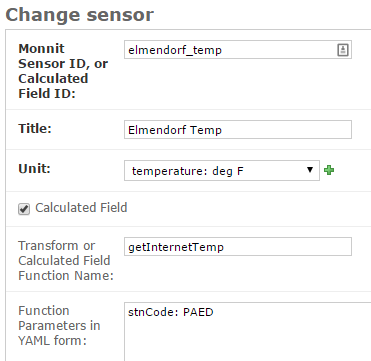In the first box, a Sensor ID has been created, in this example: `elmendorf_temp`. `Title` and `Unit` entries are filled out as they are for standard sensors. The `Calculated Field` box must be checked. For gathering outdoor dry-bulb temperature, the `Transform or Calculated Field Function Name` must contain the value `getInternetTemp` (correct capitalization is critical and must be as shown). Finally, the `Function Parameters in YAML form` box must have an entry of `stnCode:` plus a 4 character National Weather Service station code, in this example (there must be a space after the colon):

```stnCode: PAED
```

The only changes necessary to acquire a wind speed value in miles per hour is to enter `getInternetWindSpeed` into the `Transform or Calculated Field Function Name` box, change the `Unit` to `velocity: mph`, and enter an appropriate Sensor ID and Title. Acquiring relative humidity data in % RH requires entering `getInternetRH` into the`Transform or Calculated Field Function Name` box, and making appropriate unit and title changes elsewhere.

The MesonetAPI service includes a larger set of weather stations. To gather temperature or wind data from this service, you must first acquire a Mesonet API Token and enter that key into the BMON Settings File as the `BMSAPP_MESONET_API_TOKEN` setting (restarting the Django web application after changing a setting is necessary).

There is currently no charge for limited use of the API up to 5,000 requests and 5 million service units per month. Beyond that there is charge of 5 cents per thousand requests, and 15 cents per million Service Units. If either your Requests or Service Units exceed the free tier levels, you will be charged a \$5.00 monthly service fee, in addition to the rated charges for any usage above the free tier levels. See the Mesonet Pricing Page for more information.

Here is an example configuration for acquiring temperature data from the service:

Calculated Field
Transform or
Calculated Field
Function Name:
`getAllMesonetTemperature`
Function
Parameters in
YAML form:
`stn: F2072`
`request_interval_hours: 2`
`since: 6/1/2019`

The key differences from the National Weather Service configuration are:

• `getAllMesonetTemperature` must be entered into the `Transform or Calculated Field Function Name` box. If you are acquiring wind speed data, then the correct entry is `getAllMesonetWindSpeed`. Capitalization must be as shown.
• The `Function Parameters` box must contain a `stn` entry for the weather station you want data from. To find station codes, refer to the Mesonet map.
• The `Function Parameters` box may contain an additional entry for the `request_interval_hours` which specifies the minimum interval at which data is updated. To stay within the limit of 5,000 requests per month, the interval can be 0.5 for up to three calculated sensors or 2.0 for up to 13. To estimate the minimum interval you can take the total number of fields that will use the mesonet API and multiply by 0.15. The default is two hours.
• The `Function Parameters` box may also contain an additional `since` entry which specifies the earliest date or date/time to retieve data for.

## Converting On/Off Events into Runtime Fraction¶

Some sensors record the precise time of On and Off events. An example of such a sensor is a Monnit Dry Contact sensor. This sensor posts a reading every time its two contacts are closed or are opened, and the sensor is often used to record when a device turns on and turns off. In addition to seeing the exact times a device turned on and turned off, it is often useful to record the percentage of time that the device was on during evenly spaced intervals.

To provide this additional information, a special Calculated Field function is provided in BMON. The function will create a separate “sensor” in the BMON system that shows the fraction of time that a device was On for every half-hour interval (or other user-configurable interval). This function is called `runtimeFromOnOff`, and here is an example of its use: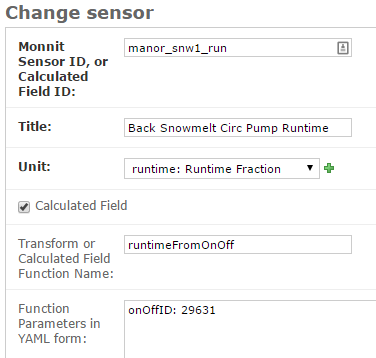The `Unit` entry generally should be `runtime: Runtime Fraction` or `fraction: Occupied Fraction`. `runtimeFromOnOff` must be entered as the `Transform or Calculated Field Function Name`. Finally, you need to provide the Sensor ID of the sensor that records the precise On and Off times (that sensor needs to report a value of 1 when the device turns on and a value of 0 when the device turns off). That Sensor ID is entered as the `onOffID` parameter in the `Function Parameters` box:

```onOffID: 29631
```

In this example, the Sensor ID is `29631`, an ID of a Monnit Dry Contact sensor. By default, this function will calculate the runtime fraction for every half-hour interval. If you would like to use a different interval, add a second line to the `Function Parameters` box. For the above example, the following would be the entry for calculating 15 minute runtime fractions:

```onOffID: 29631
runtimeInterval: 15
```

This special runtime function is also useful with Motion or Occupancy Sensors and 1-Wire Motor Sensors used with the Mini-Monitor.

## Calculating Rate of Use from a Liquid Tank such as Fuel Tank¶

Sensors are available that can determine the depth of liquid in a tank. One example of such a sensor is an ultrasonic distance measuring sensor mounted on the top of the tank looking down at the liquid in the tank. Pressure sensors can be used to determine the depth of liquid in a tank. While the depth of liquid in the tank is a useful quantity to measure and display, it is also useful to know how fast the liquid is leaving the tank; i.e. the rate-of-use of the liquid.

The `tankUse` calculated sensor function is available to create a new calculated sensor that shows the rate-of-use from a tank. The function needs two sensors to supply the data for determining rate of use:

• A sensor that reports depth of liquid in the tank in inches. For a distance-meansuring sensor mounted at the top of the tank looking downward, a sensor Transform can be entered, e.g. `39.41 - val`, that converts the distance measured to the liquid surface into a liquid depth. Determining the constant in this Transform can be done by recording a liquid depth reading from a sight glass or a dipstick at a time when a sensor reading of the distance to liquid surface is available. The 39.41 value in the prior formula was determined by adding a dipstick reading of 22.25 inches to a distance-to-liquid reading of 17.16 inches.
• A sensor that measures the temperature near the tank, or on the tank wall. Liquids expand and contract with temperature, and some sensors themselves change their readings with temperature, so the `tankUse` function uses the temperature readings to factor out temperature impacts on the liquid depth measurement.

The screenshot below shows a basic use of the `tankUse` function: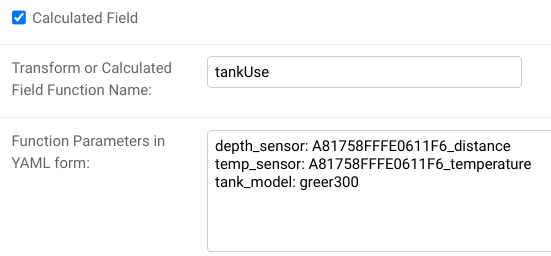The `depth_sensor` and `temp_sensor` parameters are required and give the Sensor IDs of the two sensors previously described. The depth sensor must report inches of liquid depth. The temperature sensor can report in any units and be located on or near the tank (on the tank is most accurate.) The `tank_model` parameter identifies the model of tank being measured. Only certain Greer tanks are known by the function, but other tanks can be modeled through use of tank geometry parameters described below. As set up above, this function will report daily-average BTU/hour of usage from the tank, assuming the tank contains #1 Heating Oil.

Note that the most recent data points created by the function will be updated in subsequent runs of the function to improve accuracy. Estimating rate-of-use from a tank has better accuracy when data before and after the reporting period is available.

Here is the full list of available parameters for the function:

`depth_sensor` Parameter, required

The Sensor ID of the liquid depth sensor, which must report in inches.

`temp_sensor` Parameter, required

The Sensor ID of the temperature sensor, mounted on or in the tank (most accurate), or near the tank. The sensor can report in any units.

`tank_model` Parameter, optional

The function needs to know the size and shape of the tank. Certain models of tanks are known by the function, and if your tank is one of those, this parameter can be used to identify the tank model. A number of Above Ground Single Wall UL 142 tanks from Greer are known by the function. The `tank_model` ID and tank model of known tanks are shown below:

• `greer300`: 300 gallon Greer tank (horizontal, cylindrical)
• `greer500`: 500 gallon Greer tank (horizontal, cylindrical)
• `greer1000`: 1,000 gallon Green tank (horizontal, cylindrical)
• `greer1500`: 1,500 gallon Green tank (horizontal, cylindrical)

If you do not specify a `tank_model`, you must specify both a `tank_gallons` and a `tank_max_depth` parameter, described below.

`tank_gallons` Parameter, optional

If `tank_model` is not specified, you must provide the `tank_gallons` parameter, giving the tank capacity measured in gallons.

`tank_max_depth` Parameter, optional

If `tank_model` is not specified, you must provide the `tank_max_depth` parameter. The `tank_max_depth` parameter gives the liquid depth that occurs when the tank is full. The parameter must be expressed in inches.

`linear` Parameter, optional, default value: `False`

If `linear` is set to False, the default, the tank is assumed to be a horizontal, cylindrical tank. If `linear` is True, then the volume of liquid in the tank is assumed to be directly proportional to depth of liquid; this is true for vertical, cylindrical and rectangular tanks. In general, it is true for any tank where the horizontal cross-sectional area does not change across the usable height of the tank. Note that if the `tank_model` parameter is entered, then the `linear` parameter is forcibly set to a value consistent with the chosen `tank_model`; a user-entered value is ignored.

`report_hours` Parameter, optional, default value: `24`

The function reports tank usage for fixed intervals of time. This parameter controls how long those intervals are. The default value of 24 (expressed in hours) means that usage will be reported for daily intervals. Given current sensor technology, it is recommended that `report_hours` values be set to 24 or greater. Sub-day resolution is not accurate given the temperature and noise effects experienced by tank depth sensors.

`measure` Parameter, optional, default value: `btu`

The function can report rate-of-use from the tank in two different units of measure: BTU/hour and gallons/hour. To select BTU/hour, `measure` should be set to `btu`, which is the default. To select gallons/hour, `measure` should be set to `gallon`.

`fuel_btus` Parameter, optional, default value: `137452`

If the `measure` selected is `btu`, this parameter gives the number of BTUs in a gallon of tank fuel, defaulting to 137,452 BTUs/gallon, which is appropriate for #1 Heating Oil.

## Storing the Raw Count Values from a Rate-of-Change Sensor¶

Note: this function is not needed with the current BMON release, as every counter-type sensor that uses a “rate” transform will automatically generate a second sensor that holds the cumulative count. This sensor will have the Sensor ID of the original sensor plus the suffix “_raw”.

Counter type sensors generally use a Transform function to transform the cumulative count registered by the sensor into a rate-of-change of the quantity being sensed. For example, a fuel meter will register the total cumulative gallons of fuel consumed. A Transform function is usually applied to the cumulative gallon value to convert it to a rate of use per hour or per day. See the “Pulse Counter Transforms” section on the Transform Expressions page for further information.

However, somestimes it is desirable to also store the cumulative count registered by the sensor, in addition to the rate of change. The `lastCount` calculated function described in this section meets that objective. The screenshot below shows a typical configuration of the calculated function.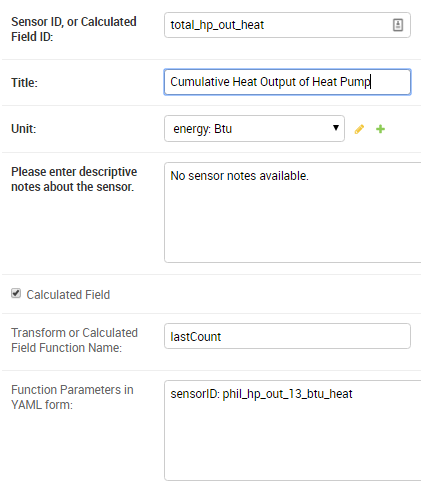The only parameter necessary is the `sensorID` (Sensor ID) of the rate-of-change sensor. This calculated function will then acquire and store the last raw count that was used by the sensor to determine the rate of change.

Note that calculated functions only run every 1/2 hour, so the time resolution for these count values will be 1/2 hour, even if the counter reports at a more frequent interval.

## Acquiring Building Energy Usage Information from ARIS¶

BMON can import building energy usage information from AHFC’s Alaska Retrofit Information System (ARIS). Configuring a sensor for the imported data is very similar to the process for acquiring weather data from the internet described above.

Using the administration interface, create a new Sensor ID. `Title` and `Unit` entries are filled out as they are for standard sensors. The `Calculated Field` box must be checked. The `Transform or Calculated Field Function Name` must contain the value `getUsageFromARIS` (correct capitalization is critical and must be as shown). Finally, the `Function Parameters in YAML form` box must have an entry of `building_id:` (there must be a space after the colon) with a valid building id number from the ARIS database, and an entry of `energy_type_id:` with a valid energy type value as described below.

Required Function Parameters in YAML form:

```building_id: 1
energy_type_id: 1
```

Additional Optional Function Parameters in YAML form:

```energy_parameter: 'EnergyQuantity'
energy_multiplier: 1
expected_period_months: 1
```

`building_id` Parameter

The easiest way to find a building_id value is to look on the ‘Commercial REAL Form’ in the ARIS user interface. When you select a building the building_id should show up in the upper left corner of the form.

`energy_type_id` Parameter

Possible values for the energy_type_id parameter:

• 1 Electric
• 2 Natural Gas
• 3 Propane
• 6 Coal
• 7 Demand - Electric
• 8 Demand - Nat Gas
• 10 Steam District Ht
• 11 Hot Wtr District Ht
• 12 Spruce Wood
• 13 Birch Wood
• 14 #1 Fuel Oil
• 15 #2 Fuel Oil

`energy_parameter` Optional Parameter

The energy_parameter specifies which value will be read from the ARIS database:

• EnergyQuantity: The amount of energy used
• DollarCost: The cost of energy for the given month
• DemandUse: The amount of energy demand
• DemandCost: The cost of energy demand for the given month, in dollars

A value of ‘EnergyQuantity’ will be used by default if you don’t include this parameter.

`energy_multiplier` Optional Parameter

The energy_multiplier is a multiplier that is used to scale the value that is read from the ARIS database. If you don’t include the parameter, a value of 1.0 will be used by default. The value that is stored is calculated as:

• For EnergyQuantity: `[stored value] = [value from ARIS] * energy_multiplier / [total hours in the read period]`
• For Costs: `[stored value] = [value from ARIS] * energy_multiplier / [standard length months in the read period]`
• For DemandUse: `[stored value] = [value from ARIS] * energy_multiplier`

`expected_period_months` Optional Parameter

In rare cases where the normal read period for the energy usage is something other than one month, you can enter a different number of months using this parameter. This value is used for estimating the previous read date when the date wasn’t set for the previous entry in ARIS, and for detecting missing data when the previous read date is more than 1.75 * [expected period months] earlier than the current read date.

To use the BMON ARIS functionality you need to enter the URL, Username and Password in your installation’s settings.py file. The required settings parameters are:

• `BMSAPP_ARIS_URL`
• `BMSAPP_ARIS_USERNAME`
• `BMSAPP_ARIS_PASSWORD`

## Estimating Pellet Consumption and Heat Output of an Okofen Pellet Boiler¶

A Periodic Script is available to collect data from Okofen Wood Pellet Boilers. One of the Sensors indicates the Status of the boiler (the P241 sensor). If the Boiler Status is in state 5 or 6, then the boiler is firing, consuming pellets, and producing heat. A special calculated field has been created, `OkoValueFromStatus`, that allows you to create a new field showing the pellet consumption rate or the heat output rate of the boiler for every 5 minute interval. Here is an example of the function in use: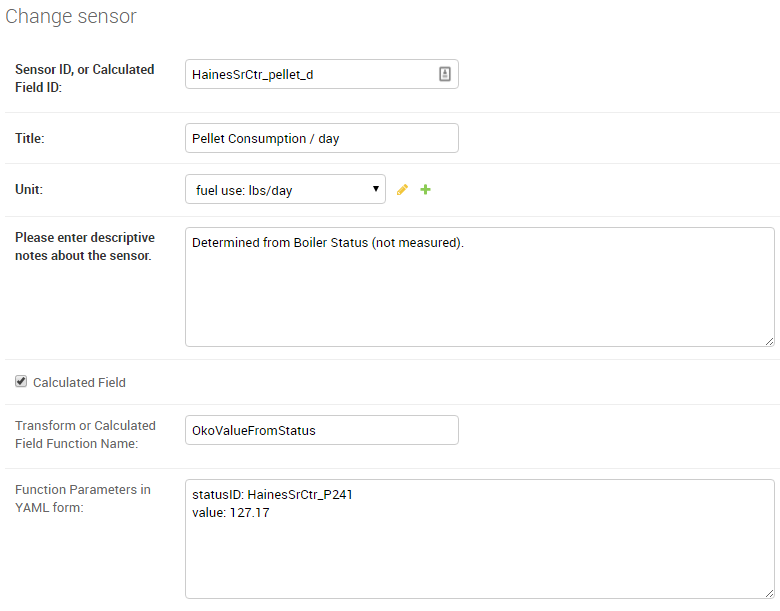There are the two critical parameters that should be provided for the function, shown here with example values:

```statusID: HainesSrCtr_P241
value: 127.17
```

The `statusID` parameter gives the Sensor ID of the boiler’s Status sensor. For the example, the Sensor ID is `HainesSrCtr_P241`. When this sensor reads a value of 5 or 6, the Okofen boiler is firing.

The `value` parameter is the pellet consumption rate or heat output rate that occurs when the boiler is firing. For this example, that rate is 127.17 pounds per day of pellets (the units were specified in the `Unit` entry of the sensor).

The calculated field will generate pellet consumption rates or heat output rates for each 5 minute interval spanning the available Status data set. It is often useful to the use the `Data Averaging` feature of the `Plot Sensor Values over Time` graph to see the average rates across day, week, or monthly periods.

## Deprecated Calculated Field Functions¶

Warning

Deprecated functions are described below and are present for backward compatibility. Instead, use the `genericCalc` feature, described earlier in this document, for new work.

Prior to development of the `genericCalc` function described above, calculated fields were only possible for a few different types of mathematical expressions. These specific types of calculated fields are described in this section, however, the `genericCalc` approach should be used in their place; the functions below are left available for backward compatibility reasons. The table below shows these functions and use of the functions is explained in the section following the table.

Function Name Expression Performed
linear
`slope * val + offset`

`slope` default is 1.0
`offset` default is 0.0
AminusB
`A - B`
AplusBplusCplusD
`A + B + C + D`

`C` default is 0.0
`D` default is 0.0
fluidHeatFlow
`flow * (Thot - Tcold  * multiplier * (1.0-heat_recovery)`

`heat_recovery` default is 0.0

Each one of these functions can create a Calculated Field based by applying a mathematical expression to a number of variables. The mathematical expression that is used is shown in the `Expression Performed` column of the table above. Each expression has a number of variables. Each variable can either be a number or Sensor ID (at least one of the variables must be a Sensor ID). Variables may have default values, as indicated in the table above. If a variable has a default value, it does not need to appear in the `Function Parameters` configuration box. Here is an example for the `linear` function: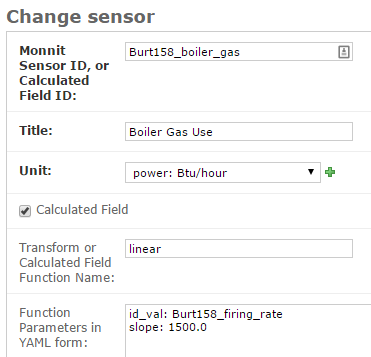In this example, there already is a sensor that reports the firing rate of a boiler as a percentage value varying from 0 to 100. We now want to create a Calculated Field that displays the rate of natural gas use of the boiler, expressed in Btu/hour. Because the gas use and the firing rate of the boiler are linearly related, we can use the `linear` Calculated Field function to create this gas usage field. Multiplying the firing rate by 1500 will give the gas usage in Btu/hour since the maximum gas usage of the boiler is 150,000 Btu/hour; a 100 firing rate times 1500 gives a gas usage of 150,000.

The `linear` function has three variables: `val`, `slope`, and `offset`. For our example, our conversion multiplier of 1500 is the `slope` variable, and you can see its entry in the `Function Parameters` in the above screenshot. The `offset` variable is not needed in this application; BMON has a default value of 0.0 for this variable, which is correct for our application, so therefore we need not provide the variable in the `Function Parameters` box. Finally, the `val` variable will be used for the Firing Rate sensor values that we are using to calculate gas usage. Since this variable needs to be filled in with sensor values, we need to preface the variable with ``id_`` to indicate that this variable is a set of sensor values. Then, the value provided for the variable in the `Function Parameters` box is a Sensor ID:

```id_val: Burt158_firing_rate
```

The `id_` prefix on the variable `val` indicates that the variable will be taken from an existing sensor. `Burt158_firing_rate` is the Sensor ID of the firing rate sensor.

So, every 30 minutes BMON will gather up all of the `Burth158_firing_rate` sensor readings that have not already been used previously in this calculation, and BMON will multiply the by 1500 to create additional sensor readings for the `Burt158_boiler_gas` sensor.

Here is a more complicated example that creates a Calculated Field that estimates the natural gas usage of a sidewalk snowmelt system based on measuring supply and return temperatures and the runtime of a circulating pump: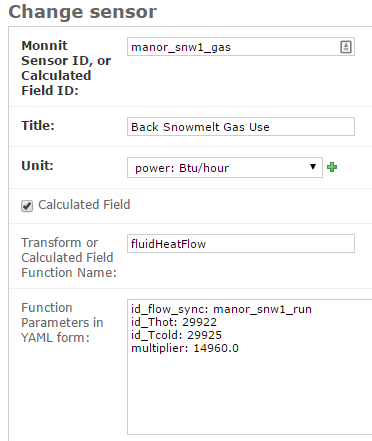The Calculated Function being used here is the `fluidHeatFlow` function, as described in the table above. You can see in the `Function Parameters` box that the `heat_recovery` variable is not provided in the configuration of this Calculated Field. Therefore, the `heat_recovery` variable will assume its default value of 0.0. Three of the variables in the math expression for the `fluidHeatFlow` function come from existing sensor values: `flow`, `Thot`, and `Tcold`. In the `Function Parameter` box, these variable names are prefaced by the `id_` prefix, indicating the values provided are Sensor IDs. The `multiplier` variable is not a sensor value but instead the constant 14960.0.

Finally, you can see that the `flow` variable appears in the `Function Parameter` box as `id_flow_sync`. As explained before, the `id_` prefix indicates that the variable comes from a Sensor. The `_sync` suffix indicates that the final calculated values for the new sensor (`manor_snw1_gas`) should be synchronized on the timestamps of this sensor. The other input sensor values (`Thot` and `Tcold`) will be interpolated to these timestamp values when the calculation occurs. If you have multiple sensor values entering into a Calculated Field, you can add the suffix `_sync` to the variable whose timestamp values should be used for the resulting calculated values. If you do not append `_sync` to one of the variable names, one of the inputs sensors will be used for synchronization, but it will not be easy to determine which one.